# Google Sheets: everyday formulas examples

Spreadsheets offer a great platform to manage data tables. But are there any easy Google Sheets functions for daily calculations? Find out below.

I believe the most required operation in tables is to find the total sum of different values. The first thing that comes to mind is to add every single cell of interest:

`=E2+E4+E8+E13`

But this formula will become extremely time-consuming if there are too many cells to take into account.

The proper way to add cells is to use a special Google Sheets function – SUM – that lists all cells automatically using commas:

`=SUM(E2,E4,E8,E13)`

If the range consists of adjacent cells, simply indicate its first and last cells even if there are blank ones somewhere between. Thus, you'll avoid enumerating each and every cell in Google Sheets SUM formula.

Tip. Another way to add SUM is to select the column with numbers and pick SUM under the Formulas icon: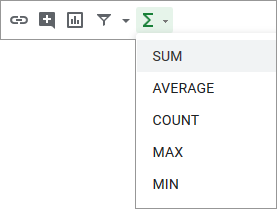The result will be inserted to a cell right below the selected range.

Tip. Our Power Tools has an AutoSum feature. One click – and your active cell will return the sum of values from the entire column above.

Let me complicate the task. I want to add numbers from different data ranges on multiple sheets, for example, A4:A8 from Sheet1 and B4:B7 from Sheet2. And I want to sum them in a single cell:

`=SUM('Sheet1'!A4:A8,'Sheet2'!B4:B7)`

As you can see, I just added one more sheet into Google Sheets SUM formula and separated two different ranges by a comma.

## Percentage formulas

I often hear people ask about finding the percentage of different totals. This is usually calculated by the Google Sheets percentage formula like this:

=Percentage/Total*100

The same also works whenever you need to check what part this or that number represents of the total:

=Part/Total*100

Tip. Master percentage of a total, total & amount by percent, its increase & decrease in this tutorial.

In my table where I keep records of all sales for the past 10 days, I can calculate the percentage of each sale from the total sales.

First, I go to E12 and find total sales:

`=SUM(E2:E11)`

Then, I check what part the first day sales constitutes of the total in F2:

`=E2/\$E\$12`

I recommend to make a few adjustments as well:

1. Turn E2 to an absolute reference\$E\$12 – to make sure you divide each day's sale by the same total.
2. Apply the percent number format to cells in column F.
3. Copy the formula from F2 to all cells below – up to F11.

Tip. To copy the formula, use one of the ways I mentioned earlier.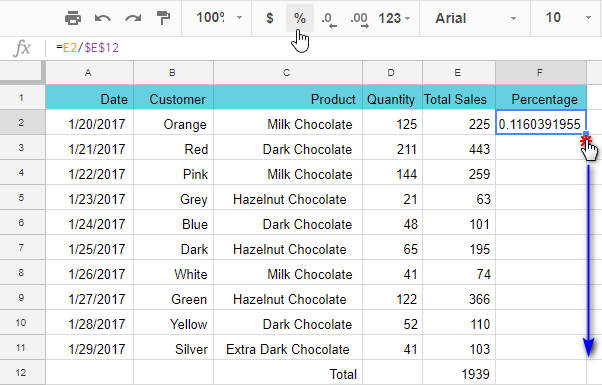Tip. To make sure your calculations are correct, enter the below one to F12:

`=SUM(F2:F11`)

If it returns 100% – everything's correct.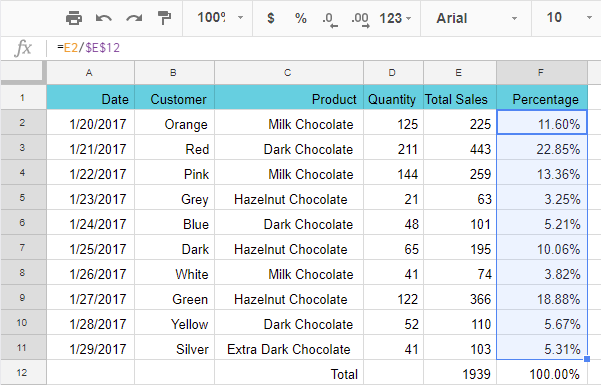Why do I recommend using the percentage format?

Well, on one hand, to avoid multiplying each result by 100 if you'd like to get percents. On the other, to avoid dividing the results to 100 if you want to use them for any further non-percent math operations.

Here's what I mean: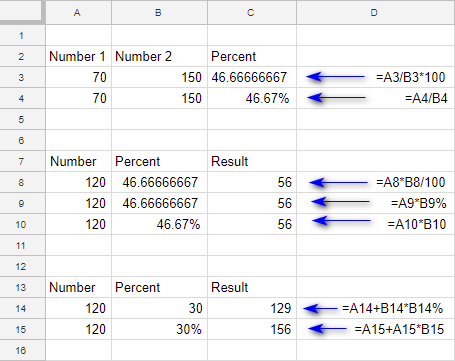I use the percentage number format in cells C4, B10, and B15. All Google Sheets formulas that reference these cells are much easier. I don't have to divide by 100 or add the percent symbol (%) to formulas in C10 and C15.

The same cannot be said about C8, C9, and C14. I must make these extra adjustments to get the correct result.

## Array formulas

To work with loads of data in Google Sheets, nested functions and other more complicated calculations are used as a rule. Array formulas are there in Google Sheets for that purpose as well.

For instance, I have a table of sales per client. I'm curious to find the maximum sale of milk chocolate to Smith to check if I can give him an extra discount next time. I use the next array formula in E18:

`=ArrayFormula(MAX(IF((\$B\$2:\$B\$13="Smith")*(\$C\$2:\$C\$13="Milk Chocolate"),\$E\$2:\$E\$13,"")))`

Note. To finish any array formula in Google Sheets, press Ctrl+Shift+Enter rather than simply Enter.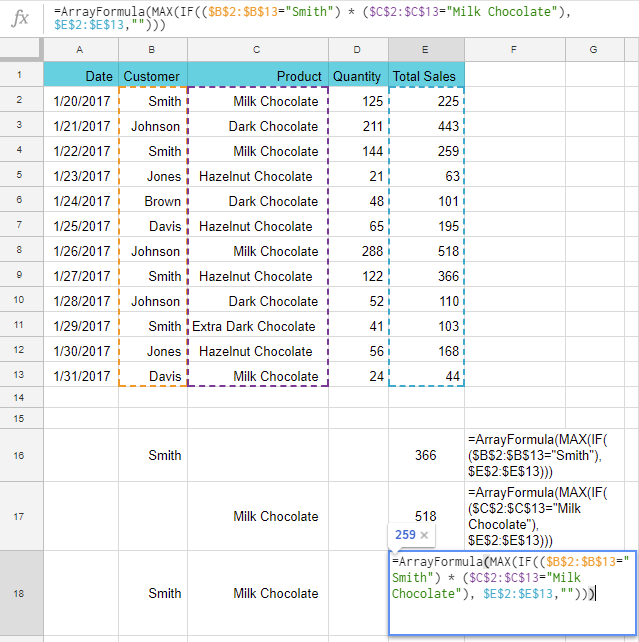I've got \$259 as a result.

My first array formula in E16 returns the maximum purchase made by Smith – \$366:

`=ArrayFormula(MAX(IF((\$B\$2:\$B\$13="Smith"),\$E\$2:\$E\$13)))`

E17 show the maximum money spent for milk chocolate – \$518:

`=ArrayFormula(MAX(IF((\$C\$2:\$C\$13="Milk Chocolate"),\$E\$2:\$E\$13)))`

Now, I'm going to replace all values used in Google Sheets formulas with their cell references: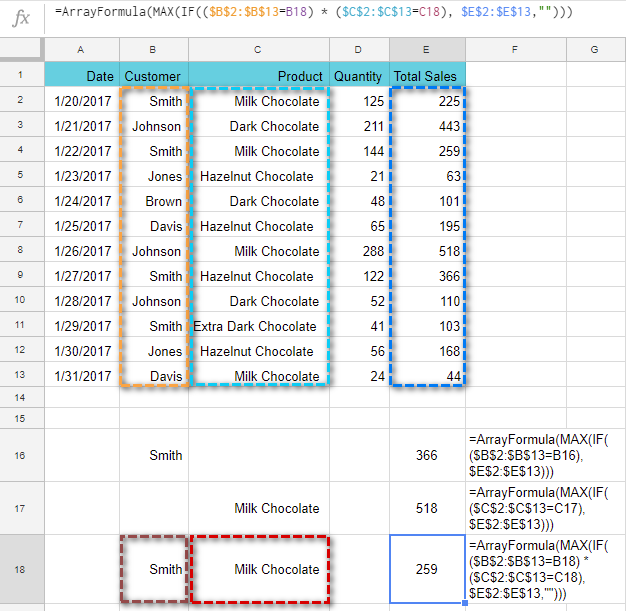Have you noticed what has changed?

`=ArrayFormula(MAX(IF((\$B\$2:\$B\$13=B18)*(\$C\$2:\$C\$13=C18),\$E\$2:\$E\$13,"")))`

`=ArrayFormula(MAX(IF((\$B\$2:\$B\$13="Smith")*(\$C\$2:\$C\$13="Milk Chocolate"),\$E\$2:\$E\$13,"")))`

Just like that, juggling with values in cells you reference you can quickly get different results based on different conditions without changing the formula itself.

## Google Sheets formulas for daily use

Let's have a look at a few more functions and formulas examples handy for every-day use.

### Example 1

Suppose your data is written partly as numbers and partly as text: 300 euros, total – 400 dollars. But you need to extract numbers only.

I know just a function for that:

=REGEXEXTRACT(text, regular_expression)

It pulls the text by mask with a regular expression.

• text – it can be cell reference or any text in double quotes.
• regular_expression – your text mask. Also in double quotes. It lets you create almost any text scheme possible.

The text in my case is a cell with a data (A2). And I use this regular expression: [0-9]+

It means that I'm looking for any quantity (+) of numbers from 0 to 9 ([0-9]) written one after another: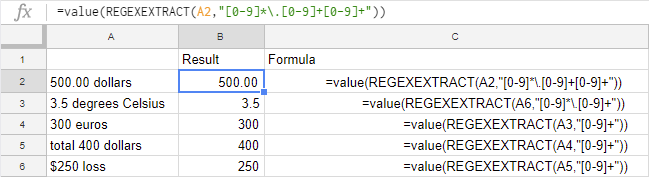If numbers has fractions, the regular expression will look like this:

`"[0-9]*\.[0-9]+[0-9]+"` for numbers with two decimal places

`"[0-9]*\.[0-9]+"` for numbers with one decimal place

Note. Google Sheets sees the extracted values as text. You need to convert them to numbers with the VALUE function or with our Convert tool.

### Example 2 – concatenate text with a formula

Formulas within the text help getting a neatly looking row with some totals – numbers with their short descriptions.

I'm going to create such rows in lines 14 and 15. To start with, I merge cells in those rows via Format > Merge cells and then count the sum for column E:

`=SUM(E2:E13)`

Then I put the text I'd like to have as a description to double quotes and combine it with the formula using an ampersand:

`="Total chocolate sales: "&SUM(E2:E13)&" dollars"`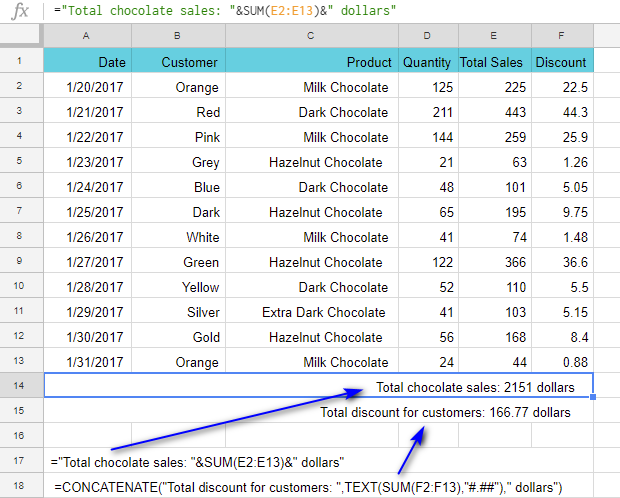To make my numbers decimals, I use the TEXT function and set the format: "#,##0"

Another way is to use Google Sheets CONCATENATE function, like I used in A15:

`=CONCATENATE("Total discount for customers: ",TEXT(SUM(F2:F13),"#.##")," dollars")`

### Example 3

What if you upload the data from somewhere and all numbers appear with spaces, like 8 544 instead of 8544? Google Sheets will return these as text, you know.

Here's how to turn these values written as text to "normal numbers":

`=VALUE(SUBSTITUTE("8 544"," ",""))`

or

`=VALUE(SUBSTITUTE(A2," ",""))`

where A2 contains 8 544.

How does it work? The SUBSTITUTE function replaces all spaces in the text (check the second argument – there's space in double-quotes) with an "empty string" (the third argument). Then, VALUE converts text to numbers.

### Example 4

There are some Google Sheets functions that help manipulate text in your spreadsheets, for instance, change the case to sentence case. If you have something strange like soURcE dAtA, you can get Source data instead: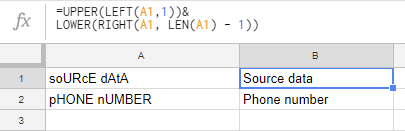Let me explain that in detail. I take the first character in a cell:

`=LEFT(A1,1)`

and switch it to the upper case:

`=UPPER(LEFT(A1,1))`

Then I take the remaining text:

`=RIGHT(A1,LEN(A1)-1)`

and force it into lower case:

`=LOWER(RIGHT(A1,LEN(A1)-1))`

Lastly, I bring all pieces of the formula together with an ampersand:

`=UPPER(LEFT(A1,1))&LOWER(RIGHT(A1,LEN(A1)-1))`

Tip. You can switch between cases in a click with the corresponding utility from our Power Tools.

Of course, there's much more Google Sheets has to offer. Don't be afraid of different complex formulas – just try and experiment. After all, these toolsets let us solve many different tasks. Good luck! :)

## You may also be interested in

1. I need a function or formula that will add a certain amount of money to the amount that is already in the cell daily and automatically so that I know how much someone owes me daily. It would be \$0.10 added daily.

• Hello Les,

If you want to change the amount in the same cell where it's written, you need to use Google Apps Script. You will find a lot of helpful content and links here: https://developers.google.com/apps-script/overview

If you're okay with having the result in a neighboring cell, you can try this formula (assuming your amount in A2):
=SUM(A2,0.1*DATEDIF("1/08/2022",today(),"d"))

2. hi
following there are 3 coulumn "A B C,,

A B C
-www.ro.com Gohan 2501
-www.ro.com Giyan 2500
-www.ro.com Giyan 908
-www.ui.com Gohan 9801
-www.ui.com Lisa 9802
-www.ui.com Lisa 9803
-www.uip89.com Lisa 9864
-www.uip67.com Lisa 9834
-www.uipo.com Gohan 9805
-www.uipo.com Gohan 9806

condition is going to very complicated
I need "max" value of (C) column, where B=Lisa
but i need lisa ---> unique links --> -www.ui.com, so should be dispaly this value, sum range--> 9802 + 9803=19605,
i know it could be possible with Maxif formula but there are thousand of links where so many duplicates values, i just need to find max value from sum range of uniquelinks,
is there any formula who automatically select sum of (Column C) from each unique links which is Group by Lisa,
formula should be consider sum of every unique (links) value and find max value,
i.e
lisa----> unique ---> http://www.uip89.com 9864
lisa----> unique ---> http://www.uip67.com 9834
Lisa---->unique--> -www.ui.com 9802 + 9803=19605,
so the max value is 19605,,, how it could be display with max formula condition with these condition

• Hi Saha,

I'm sorry, I don't understand why in your example you sum 9802 & 9803 if the link for those numbers appears twice (it's not unique). If I'm missing something, please try to explain it in more detail.

• suppose here-- > http://www.ui.com repeated twice where http://www.ui.com = 9864 and
http://www.ui.com = 9803, here two Amount (9864 &9803) Raised in same link ----> http://www.ui.com
(Both amount (9864 &9803) Raised on diffrent days so its shown twice entry in excell sheet )
means total Sum Amount of Rs.19605 Raised in this link " http://www.ui.com "
there are many links are Shown as twice&thrice or four time because Amount Raised on diffrent days, or from diffrent person,

so i just need a formula where it should be Calculate cumulative Value of (sum of raised amount) each unique links, it shoulbe be show all unique link with sum of each Raised Amount,
http://www.ui.com =19605 (two times Amount raised in this link)
http://www.ro.com =3300 (three time Amount raised in this link)
http://www.fuf.com = 4404

and find
max value i.e (19605) from the all unique links,,

(sorry , please avoide my all grammer mistake, cause i'm useless and i dont know how to write and speak english)

• Thank you, Saha.

Here's a formula for you:
=QUERY({QUERY({A1:C11},"select Col1, Col2, SUM(Col3) group by Col1,Col2")},"select Max(Col3) label Max(Col3) ''",0)

But I should mention that it's for Google Sheets (as is the blog post). In your second comment, you mentioned that you have an Excel sheet. If so, please specify because this formula is for Google Sheets only.

3. Dear Sir,

• Dear Ravinder,

It's Ablebits. Look for a YouTube icon at the very bottom of our website, there's a link on every page :)

4. i have 34 excel sheet in a file and have same colomn and heading and want to merge data in one sheet

• Hello Khalid,

You will find the easiest way to combine data in Excel described in another blog post.

5. how to micro run in google sheet?
how to connect excel sheet to google sheet automatically update.

• Hello Prikshit,

You will find the info on how to use macros in Google Sheets in this Docs Editors Help page.